Test 4 Section 3 #14

When you’re dividing complex numbers, you have to multiply the top and bottom of the fraction by the complex conjugate of the bottom. This creates a real number in the bottom of the fraction, which is awesome.

The bottom of this fraction is, so its complex conjugate is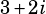. To get started, then, we write the following:Then we simplify as much as we can. First we FOIL: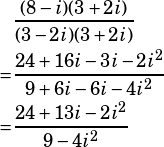Now, remembering that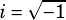and therfore that, we make that substitution and simplify further: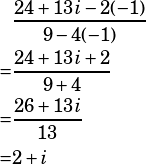So there you have it. Once we simplify that complex fraction intoform, we see that it’s just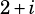, which means.